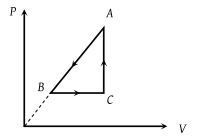P-V diagram of a cyclic process ABCA is as shown in figure. Choose the correct statement(1) $\Delta {Q}_{A\to B}$ = negative

(2) $\Delta {U}_{B\to C}$ = positive

(3) $\Delta {W}_{CAB}$ = negative

(4) All of these

Concept Videos :-

#29 | Work done in Cyclic Process
#30 | Solved Example: 13

Concept Questions :-

Cyclic process